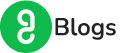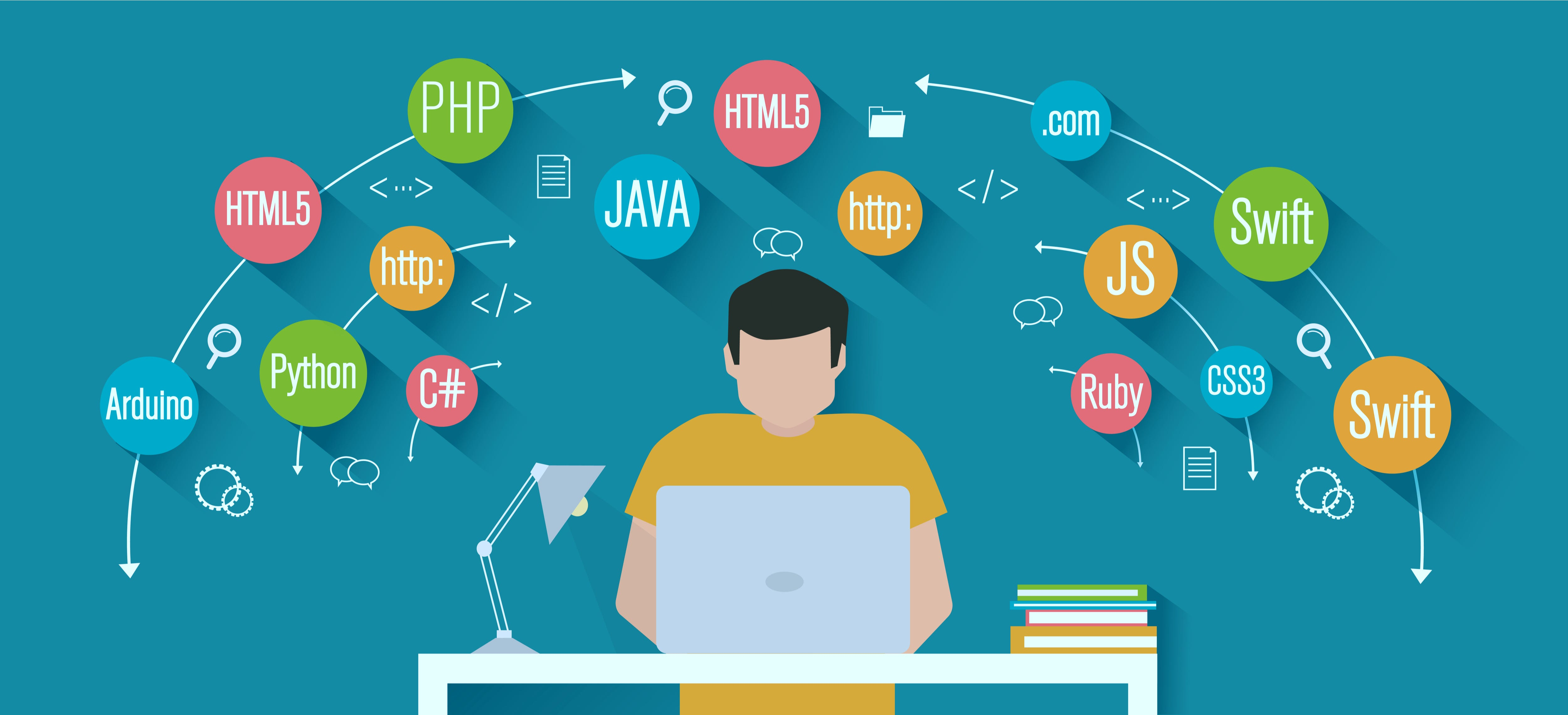# Top 6 Essential Prerequisites For Machine Learning

What are the prerequisites for Machine Learning?

Have you ever wondered how your google assistant plays songs on your demand? How do self-driving cars work? How are online recommendations in Google and Netflix catered for you? The answer is simple: Machine learning.

We live in a world where algorithms surround us for better and for worse. Moreover, every sector has benefitted from the rapid incorporation of machine learning algorithms.  Machine learning is a subset of artificial intelligence (AI). It focuses on building applications that learn from data. They even improve their accuracy without being programmed.

In simple words, ML works on the premise that machines (algorithms), given enough time and experience, will think and make decisions. These decisions are based on given choices, with past experiences. This happens in a completely automated way. More like human beings. Sounds exciting, right?

## Top 6 Essential Prerequisites for Machine Learning

Without any further delay, let us get started with the top 6 essential prerequisites for machine learning!

Diving into machine learning can be a daunting task. However, mastering the essential prerequisites of machine learning eases the process. That is to say, there are various prerequisites for machine learning.

### #1 Statistics

Firstly, statistics is the science of collecting, observing, analyzing, and interpreting meaningful information from the raw data.

We try to simplify massive data, figure out patterns. In the process, we try to answer common questions. For example:

• Name the most common data value or expected observation?
• What are the upper and lower limits on the observations?
• Explain the type of data?
• Is there any pattern in the data?

Statistical methods are must-required to understand the data used to train a machine learning model. Also, to find the results of testing different machine learning models.Thus, it is considered a basic prerequisite required for machine learning.

There are two types of statistics

1. Descriptive Statistics
2. Inferential Statistics.

#### Descriptive Statistics

As its name suggests,  descriptive statistics explain a particular dataset used to summarize the dataset into something more meaningful.

#### Inferential Statistics

Secondly, inferential statistics conclude from a sample instead of the whole dataset, focusing on individual data elements.

AI Concepts and statistics are two closely related fields of study. So much so that statisticians refer to machine learning as “applied statistics” or “statistical learning.” And not the computer-science-centric name.

#### Topics you need to familiarize on Statistics

Here are some crucial topics in statistics you need to focus on:

• Mean
• Median
• Standard deviation
• Outliers
• Histogram

### #2 Probability

Probability is a field of mathematics that deals with numerical valuation of how likely an event occurs.

Probability is a measure of uncertainty. It applies to machine learning as we need to make decisions with incomplete information in the real world.

Probability helps in assessing the risk involved in tasks of the real world. With the help of probability, we can model elements of uncertainty. These uncertainties can be anything like the risk in financial transactions and many other business processes.

In contrast, in traditional programming, we work with deterministic problems, i.e., the solution is not affected by uncertainty.#### Topics you need to familiarize on Probability

In Machine Learning and AI, you must be familiar with the following topics:

• Notation
• Probability distribution (joint and conditional)
• Different rules of probability (the Bayes theorem, the sum rule, and the product/chain rule)
• Independence
• Continuous random variables

### #3 Linear Algebra

Again, Linear algebra is a field of mathematics.  It is concerned with vectors, matrices, and operations on the data structures. It is a necessary part of machine learning. As a machine learning practitioner, you must have an understanding of linear algebra. So, why is linear algebra used so much to describe machine learning algorithms?Linear algebra is about vectors and matrices. In machine learning, we are continually working with vectors and matrices (arrays) of data. Linear algebra is essentially the mathematics of data.

It provides useful shortcuts for describing data and operations on data. Also, we need to perform these operations in machine learning methods.

#### Topics you need to familiarize on Linear Algebra

Here are some vital topics in linear algebra you need to focus on:

• Algorithms in code
• Linear transforms
• Notations
• Matrix multiplication
• Tensor and the tensor rank

### #4 Calculus

Calculus is the part of mathematics. It involves the study of the rate of change of quantities, length, area, and volume of objects. The change of quantities is interpreted as slopes of curves. Here, calculus is divided into two major parts, differential and integral calculus.

Calculus, especially Multivariable Calculus, is essential for machine learning. Furthermore, in machine learning, most models are built with a data set having several features or predictors.

Hence familiarity with multivariable calculus is crucial for building machine learning models.#### Topics you need to familiarize yourself on Calculus

Some important topics to be familiar with:

• Functions of several variables
• Step function, Sigmoid function, Logit function, ReLU (Rectified Linear Unit) function
• Cost function
• Plotting of functions
• Minimum and Maximum values of a function

### #5 Programming Languages, essential Prerequisites for Machine Learning

Both Machine Learning and Artificial Intelligence algorithms are executed with codes.

While you could get away as a beginner programmer and focus more on the mathematics front. However, mastering programming language and good coding skills is the key. It helps in understanding the internal mechanism.

Though many programming languages are used to write ML and AI-based applications. However, writing every algorithm from scratch is time-consuming.So, the best programming language is the one that comes with pre-built libraries and has advanced support of data science and data models.

In Machine Learning, Python and R are two popular languages. As far as the learning is concerned, both programming languages come with terrific support online with many resources. But, out of the two, python is preferred by beginners.

#### Concepts you need to familiarize on Programming language

Primarily, you need to learn basic coding concepts like:

### #6 Exploratory Data AnalysisExploratory Data Analysis refers to the crucial process of performing initial investigations on data. These investigations could be anything like discovering patterns and spotting anomalies. Or testing hypotheses, and checking assumptions. Moreover, they can be performed with summary statistics and graphical representations.

It is good to understand the data first. Gathering as much knowledge from it is beneficial. EDA is all about making sense of data in hand before getting them dirty with it.

Learning iterative algorithms alone can cause errors in the set and model. A more in-depth insight into how data modeling functions are necessary.

## Final Words on Prerequisites for Machine Learning

Thus, working with data is an important skill one must practice to pursue machine learning as a career.

Here, we covered the essential prerequisites of Machine Learning. Also discussed the most preferred programming languages. Furthermore, we observed that mastering the prerequisites is the stepping stone to machine learning. These prerequisites of machine learning are -statistics, probability, calculus, linear algebra, and programming knowledge.

With so many things to learn, this might indeed come as a tiresome process.

But undoubtedly, getting a hang of the prerequisites makes it easy. Then the learning process of machine learning is the most straightforward and most streamlined of all.

So, start the journey with the concepts you are familiar with. Then work your way up from there!

Contact Form

By clicking 'Submit' you Agree to Guvi Terms & Conditions.

Our Trending Courses

Our Learners Work at

## Author BioRam Kumar

## Our Live Classes

### Full Stack Development Program (FSD)

Learn Javascript, HTML, CSS, Java, Data Structure, MongoDB & more

### IIT-M Advanced Programming & Data Science Program

Learn Python, Machine Learning, NLP, Tableau, PowerBI & more

### Automation and Testing Program

Learn Selenium, Python, Java, Jenkins, Jmeter, API Testing & more

### UI/UX Program

UX Processes, Design systems, Responsive UI, & more with placement assistance.

## Related Articles

If you are reading “var functionName = function and function functionName,” we can understand how confusing it is for you.